Documentation

# imuSensor

IMU simulation model

## Description

The `imuSensor` System object™ models receiving data from an inertial measurement unit (IMU).

To model an IMU:

1. Create the `imuSensor` object and set its properties.

2. Call the object with arguments, as if it were a function.

## Creation

### Syntax

``IMU = imuSensor``
``IMU = imuSensor('accel-gyro')``
``IMU = imuSensor('accel-mag')``
``IMU = imuSensor('accel-gyro-mag')``
``IMU = imuSensor(___,'ReferenceFrame',RF)``
``IMU = imuSensor(___,Name,Value)``

### Description

example

````IMU = imuSensor` returns a System object, `IMU`, that computes an inertial measurement unit reading based on an inertial input signal. `IMU` has an ideal accelerometer and gyroscope.```

example

````IMU = imuSensor('accel-gyro')` returns an `imuSensor` System object with an ideal accelerometer and gyroscope. `imuSensor` and `imuSensor('accel-gyro')` are equivalent creation syntaxes.```

example

````IMU = imuSensor('accel-mag')` returns an `imuSensor` System object with an ideal accelerometer and magnetometer.```

example

````IMU = imuSensor('accel-gyro-mag')` returns an `imuSensor` System object with an ideal accelerometer, gyroscope, and magnetometer.```
````IMU = imuSensor(___,'ReferenceFrame',RF)` returns an `imuSensor` System object that computes an inertial measurement unit reading relative to the reference frame `RF`. Specify `RF` as `'NED'` (North-East-Down) or `'ENU'` (East-North-Up). The default value is `'NED'`.```

example

````IMU = imuSensor(___,Name,Value)` sets each property `Name` to the specified `Value`. Unspecified properties have default values. This syntax can be used in combination with any of the previous input arguments.```

## Properties

expand all

Unless otherwise indicated, properties are nontunable, which means you cannot change their values after calling the object. Objects lock when you call them, and the `release` function unlocks them.

If a property is tunable, you can change its value at any time.

Type of inertial measurement unit, specified as a `'accel-gyro'`, `'accel-mag'`, or `'accel-gyro-mag'`.

The type of inertial measurement unit specifies which sensor readings to model:

• `'accel-gyro'` –– Accelerometer and gyroscope

• `'accel-mag'` –– Accelerometer and magnetometer

• `'accel-gyro-mag'` –– Accelerometer, gyroscope, and magnetometer

You can specify `IMUType` as a value-only argument during creation or as a `Name,Value` pair.

Data Types: `char` | `string`

Sample rate of the sensor model in Hz, specified as a positive scalar.

Data Types: `single` | `double`

Operating temperature of the IMU in degrees Celsius, specified as a real scalar.

Tunable: Yes

Data Types: `single` | `double`

Magnetic field vector in microtesla, specified as a three-element row vector in the local navigation coordinate system.

The default magnetic field corresponds to the magnetic field at latitude zero, longitude zero, and altitude zero.

Tunable: Yes

Data Types: `single` | `double`

Accelerometer sensor parameters, specified by an `accelparams` object.

Tunable: Yes

Gyroscope sensor parameters, specified by a `gyroparams` object.

Tunable: Yes

Magnetometer sensor parameters, specified by a `magparams` object.

Tunable: Yes

Random number source, specified as a character vector or string:

• `'Global stream'` –– Random numbers are generated using the current global random number stream.

• `'mt19937ar with seed'` –– Random numbers are generated using the mt19937ar algorithm with the seed specified by the `Seed` property.

Data Types: `char` | `string`

Initial seed of an mt19937ar random number generator algorithm, specified as a real, nonnegative integer scalar.

#### Dependencies

To enable this property, set `RandomStream` to `'mt19937ar with seed'`.

Data Types: `single` | `double` | `int8` | `int16` | `int32` | `int64` | `uint8` | `uint16` | `uint32` | `uint64`

## Usage

### Syntax

``[accelReadings,gyroReadings] = IMU(acc,angVel)``
``[accelReadings,gyroReadings] = IMU(acc,angVel,orientation)``
``[accelReadings,magReadings] = IMU(acc,angVel)``
``[accelReadings,magReadings] = IMU(acc,angVel,orientation)``
``[accelReadings,gyroReadings,magReadings] = IMU(acc,angVel)``
``[accelReadings,gyroReadings,magReadings] = IMU(acc,angVel,orientation)``

### Description

````[accelReadings,gyroReadings] = IMU(acc,angVel)` generates accelerometer and gyroscope readings from the acceleration and angular velocity inputs.This syntax is only valid if `IMUType` is set to `'accel-gyro'` or `'accel-gyro-mag'`.```
````[accelReadings,gyroReadings] = IMU(acc,angVel,orientation)` generates accelerometer and gyroscope readings from the acceleration, angular velocity, and orientation inputs.This syntax is only valid if `IMUType` is set to `'accel-gyro'` or `'accel-gyro-mag'`.```
````[accelReadings,magReadings] = IMU(acc,angVel)` generates accelerometer and magnetometer readings from the acceleration and angular velocity inputs.This syntax is only valid if `IMUType` is set to `'accel-mag'`.```
````[accelReadings,magReadings] = IMU(acc,angVel,orientation)` generates accelerometer and magnetometer readings from the acceleration, angular velocity, and orientation inputs.This syntax is only valid if `IMUType` is set to `'accel-mag'`.```
````[accelReadings,gyroReadings,magReadings] = IMU(acc,angVel)` generates accelerometer, gyroscope, and magnetometer readings from the acceleration and angular velocity inputs.This syntax is only valid if `IMUType` is set to `'accel-gyro-mag'`.```
````[accelReadings,gyroReadings,magReadings] = IMU(acc,angVel,orientation)` generates accelerometer, gyroscope, and magnetometer readings from the acceleration, angular velocity, and orientation inputs.This syntax is only valid if `IMUType` is set to `'accel-gyro-mag'`.```

### Input Arguments

expand all

Acceleration of the IMU in the local navigation coordinate system, specified as a real, finite N-by-3 array in meters per second squared. N is the number of samples in the current frame.

Data Types: `single` | `double`

Angular velocity of the IMU in the local navigation coordinate system, specified as a real, finite N-by-3 array in radians per second. N is the number of samples in the current frame.

Data Types: `single` | `double`

Orientation of the IMU with respect to the local navigation coordinate system, specified as a `quaternion` N-element column vector or a 3-by-3-by-N rotation matrix. Each `quaternion` or rotation matrix represents a frame rotation from the local navigation coordinate system to the current IMU sensor body coordinate system. N is the number of samples in the current frame.

Data Types: `single` | `double` | `quaternion`

### Output Arguments

expand all

Accelerometer measurement of the IMU in the sensor body coordinate system, specified as a real, finite N-by-3 array in meters per second squared. N is the number of samples in the current frame.

Data Types: `single` | `double`

Gyroscope measurement of the IMU in the sensor body coordinate system, specified as a real, finite N-by-3 array in radians per second. N is the number of samples in the current frame.

Data Types: `single` | `double`

Magnetometer measurement of the IMU in the sensor body coordinate system, specified as a real, finite N-by-3 array in microtelsa. N is the number of samples in the current frame.

Data Types: `single` | `double`

## Object Functions

To use an object function, specify the System object as the first input argument. For example, to release system resources of a System object named `obj`, use this syntax:

`release(obj)`

expand all

 `step` Run System object algorithm `release` Release resources and allow changes to System object property values and input characteristics `reset` Reset internal states of System object

## Examples

expand all

The `imuSensor` System object™ enables you to model the data received from an inertial measurement unit consisting of a combination of gyroscope, accelerometer, and magnetometer.

Create a default `imuSensor` object.

`IMU = imuSensor`
```IMU = imuSensor with properties: IMUType: 'accel-gyro' SampleRate: 100 Temperature: 25 Accelerometer: [1x1 accelparams] Gyroscope: [1x1 gyroparams] RandomStream: 'Global stream' ```

The `imuSensor` object, `IMU`, contains an idealized gyroscope and accelerometer. Use dot notation to view properties of the gyroscope.

`IMU.Gyroscope`
```ans = gyroparams with properties: MeasurementRange: Inf rad/s Resolution: 0 (rad/s)/LSB ConstantBias: [0 0 0] rad/s AxesMisalignment: [0 0 0] % NoiseDensity: [0 0 0] (rad/s)/√Hz BiasInstability: [0 0 0] rad/s RandomWalk: [0 0 0] (rad/s)*√Hz TemperatureBias: [0 0 0] (rad/s)/°C TemperatureScaleFactor: [0 0 0] %/°C AccelerationBias: [0 0 0] (rad/s)/(m/s²) ```

Sensor properties are defined by corresponding parameter objects. For example, the gyroscope model used by the `imuSensor` is defined by an instance of the `gyroparams` class. You can modify properties of the gyroscope model using dot notation. Set the gyroscope measurement range to 4.3 rad/s.

`IMU.Gyroscope.MeasurementRange = 4.3;`

You can also set sensor properties to preset parameter objects. Create an `accelparams` object to mimic specific hardware, and then set the IMU `Accelerometer` property to the `accelparams` object. Display the `Accelerometer` property to verify the properties are correctly set.

```SpecSheet1 = accelparams( ... 'MeasurementRange',19.62, ... 'Resolution',0.00059875, ... 'ConstantBias',0.4905, ... 'AxesMisalignment',2, ... 'NoiseDensity',0.003924, ... 'BiasInstability',0, ... 'TemperatureBias', [0.34335 0.34335 0.5886], ... 'TemperatureScaleFactor', 0.02); IMU.Accelerometer = SpecSheet1; IMU.Accelerometer```
```ans = accelparams with properties: MeasurementRange: 19.62 m/s² Resolution: 0.00059875 (m/s²)/LSB ConstantBias: [0.4905 0.4905 0.4905] m/s² AxesMisalignment: [2 2 2] % NoiseDensity: [0.003924 0.003924 0.003924] (m/s²)/√Hz BiasInstability: [0 0 0] m/s² RandomWalk: [0 0 0] (m/s²)*√Hz TemperatureBias: [0.34335 0.34335 0.5886] (m/s²)/°C TemperatureScaleFactor: [0.02 0.02 0.02] %/°C ```

Use the `imuSensor` System object™ to model receiving data from a stationary ideal IMU containing an accelerometer, gyroscope, and magnetometer.

Create an ideal IMU sensor model that contains an accelerometer, gyroscope, and magnetometer.

`IMU = imuSensor('accel-gyro-mag')`
```IMU = imuSensor with properties: IMUType: 'accel-gyro-mag' SampleRate: 100 Temperature: 25 MagneticField: [27.5550 -2.4169 -16.0849] Accelerometer: [1x1 accelparams] Gyroscope: [1x1 gyroparams] Magnetometer: [1x1 magparams] RandomStream: 'Global stream' ```

Define the ground-truth, underlying motion of the IMU you are modeling. The acceleration and angular velocity are defined relative to the local NED coordinate system.

```numSamples = 1000; acceleration = zeros(numSamples,3); angularVelocity = zeros(numSamples,3);```

Call `IMU` with the ground-truth acceleration and angular velocity. The object outputs accelerometer readings, gyroscope readings, and magnetometer readings, as modeled by the properties of the `imuSensor` System object. The accelerometer readings, gyroscope readings, and magnetometer readings are relative to the IMU sensor body coordinate system.

`[accelReading,gyroReading,magReading] = IMU(acceleration,angularVelocity);`

```t = (0:(numSamples-1))/IMU.SampleRate; subplot(3,1,1) plot(t,accelReading) legend('X-axis','Y-axis','Z-axis') title('Accelerometer Readings') ylabel('Acceleration (m/s^2)') subplot(3,1,2) plot(t,gyroReading) legend('X-axis','Y-axis','Z-axis') title('Gyroscope Readings') ylabel('Angular Velocity (rad/s)') subplot(3,1,3) plot(t,magReading) legend('X-axis','Y-axis','Z-axis') title('Magnetometer Readings') xlabel('Time (s)') ylabel('Magnetic Field (uT)')```Orientation is not specified and the ground-truth motion is stationary, so the IMU sensor body coordinate system and the local NED coordinate system overlap for the entire simulation.

• Accelerometer readings: The z-axis of the sensor body corresponds to the Down-axis. The 9.8 m/s^2 acceleration along the z-axis is due to gravity.

• Gyroscope readings: The gyroscope readings are zero along each axis, as expected.

• Magnetometer readings: Because the sensor body coordinate system is aligned with the local NED coordinate system, the magnetometer readings correspond to the `MagneticField` property of `imuSensor`. The `MagneticField` property is defined in the local NED coordinate system.

Use `imuSensor` to model data obtained from a rotating IMU containing an ideal accelerometer and an ideal magnetometer. Use `kinematicTrajectory` to define the ground-truth motion. Fuse the `imuSensor` model output using the `ecompass` function to determine orientation over time.

Define the ground-truth motion for a platform that rotates 360 degrees in four seconds, and then another 360 degrees in two seconds. Use `kinematicTrajectory` to output the orientation, acceleration, and angular velocity in the NED coordinate system.

```fs = 100; firstLoopNumSamples = fs*4; secondLoopNumSamples = fs*2; totalNumSamples = firstLoopNumSamples + secondLoopNumSamples; traj = kinematicTrajectory('SampleRate',fs); accBody = zeros(totalNumSamples,3); angVelBody = zeros(totalNumSamples,3); angVelBody(1:firstLoopNumSamples,3) = (2*pi)/4; angVelBody(firstLoopNumSamples+1:end,3) = (2*pi)/2; [~,orientationNED,~,accNED,angVelNED] = traj(accBody,angVelBody);```

Create an `imuSensor` object with an ideal accelerometer and an ideal magnetometer. Call `IMU` with the ground-truth acceleration, angular velocity, and orientation to output accelerometer readings and magnetometer readings. Plot the results.

```IMU = imuSensor('accel-mag','SampleRate',fs); [accelReadings,magReadings] = IMU(accNED,angVelNED,orientationNED); figure(1) t = (0:(totalNumSamples-1))/fs; subplot(2,1,1) plot(t,accelReadings) legend('X-axis','Y-axis','Z-axis') ylabel('Acceleration (m/s^2)') title('Accelerometer Readings') subplot(2,1,2) plot(t,magReadings) legend('X-axis','Y-axis','Z-axis') ylabel('Magnetic Field (\muT)') xlabel('Time (s)') title('Magnetometer Readings')```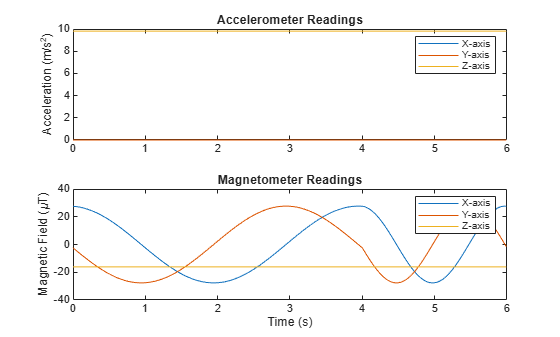The accelerometer readings indicate that the platform has no translation. The magnetometer readings indicate that the platform is rotating around the z-axis.

Feed the accelerometer and magnetometer readings into the `ecompass` function to estimate the orientation over time. The `ecompass` function returns orientation in quaternion format. Convert orientation to Euler angles and plot the results. The orientation plot indicates that the platform rotates about the z-axis only.

```orientation = ecompass(accelReadings,magReadings); orientationEuler = eulerd(orientation,'ZYX','frame'); figure(2) plot(t,orientationEuler) legend('Z-axis','Y-axis','X-axis') xlabel('Time (s)') ylabel('Rotation (degrees)') title('Orientation')```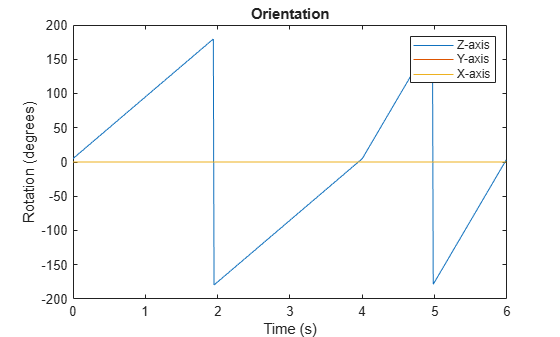Use `imuSensor` to model data obtained from a rotating IMU containing a realistic accelerometer and a realistic magnetometer. Use `kinematicTrajectory` to define the ground-truth motion. Fuse the `imuSensor` model output using the `ecompass` function to determine orientation over time.

Define the ground-truth motion for a platform that rotates 360 degrees in four seconds, and then another 360 degrees in two seconds. Use `kinematicTrajectory` to output the orientation, acceleration, and angular velocity in the NED coordinate system.

```fs = 100; firstLoopNumSamples = fs*4; secondLoopNumSamples = fs*2; totalNumSamples = firstLoopNumSamples + secondLoopNumSamples; traj = kinematicTrajectory('SampleRate',fs); accBody = zeros(totalNumSamples,3); angVelBody = zeros(totalNumSamples,3); angVelBody(1:firstLoopNumSamples,3) = (2*pi)/4; angVelBody(firstLoopNumSamples+1:end,3) = (2*pi)/2; [~,orientationNED,~,accNED,angVelNED] = traj(accBody,angVelBody);```

Create an `imuSensor` object with a realistic accelerometer and a realistic magnetometer. Call `IMU` with the ground-truth acceleration, angular velocity, and orientation to output accelerometer readings and magnetometer readings. Plot the results.

```IMU = imuSensor('accel-mag','SampleRate',fs); IMU.Accelerometer = accelparams( ... 'MeasurementRange',19.62, ... % m/s^2 'Resolution',0.0023936, ... % m/s^2 / LSB 'TemperatureScaleFactor',0.008, ... % % / degree C 'ConstantBias',0.1962, ... % m/s^2 'TemperatureBias',0.0014715, ... % m/s^2 / degree C 'NoiseDensity',0.0012361); % m/s^2 / Hz^(1/2) IMU.Magnetometer = magparams( ... 'MeasurementRange',1200, ... % uT 'Resolution',0.1, ... % uT / LSB 'TemperatureScaleFactor',0.1, ... % % / degree C 'ConstantBias',1, ... % uT 'TemperatureBias',[0.8 0.8 2.4], ... % uT / degree C 'NoiseDensity',[0.6 0.6 0.9]/sqrt(100)); % uT / Hz^(1/2) [accelReadings,magReadings] = IMU(accNED,angVelNED,orientationNED); figure(1) t = (0:(totalNumSamples-1))/fs; subplot(2,1,1) plot(t,accelReadings) legend('X-axis','Y-axis','Z-axis') ylabel('Acceleration (m/s^2)') title('Accelerometer Readings') subplot(2,1,2) plot(t,magReadings) legend('X-axis','Y-axis','Z-axis') ylabel('Magnetic Field (\muT)') xlabel('Time (s)') title('Magnetometer Readings')```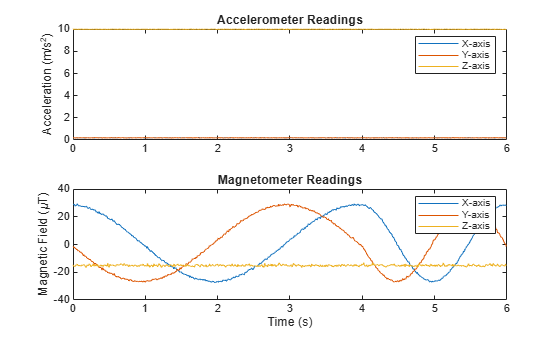The accelerometer readings indicate that the platform has no translation. The magnetometer readings indicate that the platform is rotating around the z-axis.

Feed the accelerometer and magnetometer readings into the `ecompass` function to estimate the orientation over time. The `ecompass` function returns orientation in quaternion format. Convert orientation to Euler angles and plot the results. The orientation plot indicates that the platform rotates about the z-axis only.

```orientation = ecompass(accelReadings,magReadings); orientationEuler = eulerd(orientation,'ZYX','frame'); figure(2) plot(t,orientationEuler) legend('Z-axis','Y-axis','X-axis') xlabel('Time (s)') ylabel('Rotation (degrees)') title('Orientation')```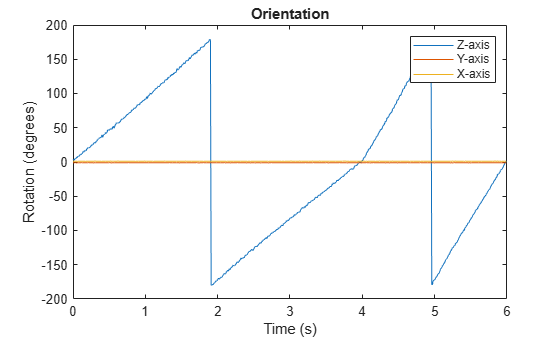`%`

Model a tilting IMU that contains an accelerometer and gyroscope using the `imuSensor` System object™. Use ideal and realistic models to compare the results of orientation tracking using the `imufilter` System object.

Load a struct describing ground-truth motion and a sample rate. The motion struct describes sequential rotations:

1. yaw: 120 degrees over two seconds

2. pitch: 60 degrees over one second

3. roll: 30 degrees over one-half second

4. roll: -30 degrees over one-half second

5. pitch: -60 degrees over one second

6. yaw: -120 degrees over two seconds

In the last stage, the motion struct combines the 1st, 2nd, and 3rd rotations into a single-axis rotation. The acceleration, angular velocity, and orientation are defined in the local NED coordinate system.

```load y120p60r30.mat motion fs accNED = motion.Acceleration; angVelNED = motion.AngularVelocity; orientationNED = motion.Orientation; numSamples = size(motion.Orientation,1); t = (0:(numSamples-1)).'/fs; ```

Create an ideal IMU sensor object and a default IMU filter object.

```IMU = imuSensor('accel-gyro','SampleRate',fs); aFilter = imufilter('SampleRate',fs); ```

In a loop:

1. Simulate IMU output by feeding the ground-truth motion to the IMU sensor object.

2. Filter the IMU output using the default IMU filter object.

```orientation = zeros(numSamples,1,'quaternion'); for i = 1:numSamples [accelBody,gyroBody] = IMU(accNED(i,:),angVelNED(i,:),orientationNED(i,:)); orientation(i) = aFilter(accelBody,gyroBody); end release(aFilter) ```

Plot the orientation over time.

```figure(1) plot(t,eulerd(orientation,'ZYX','frame')) xlabel('Time (s)') ylabel('Rotation (degrees)') title('Orientation Estimation -- Ideal IMU Data, Default IMU Filter') legend('Z-axis','Y-axis','X-axis') ```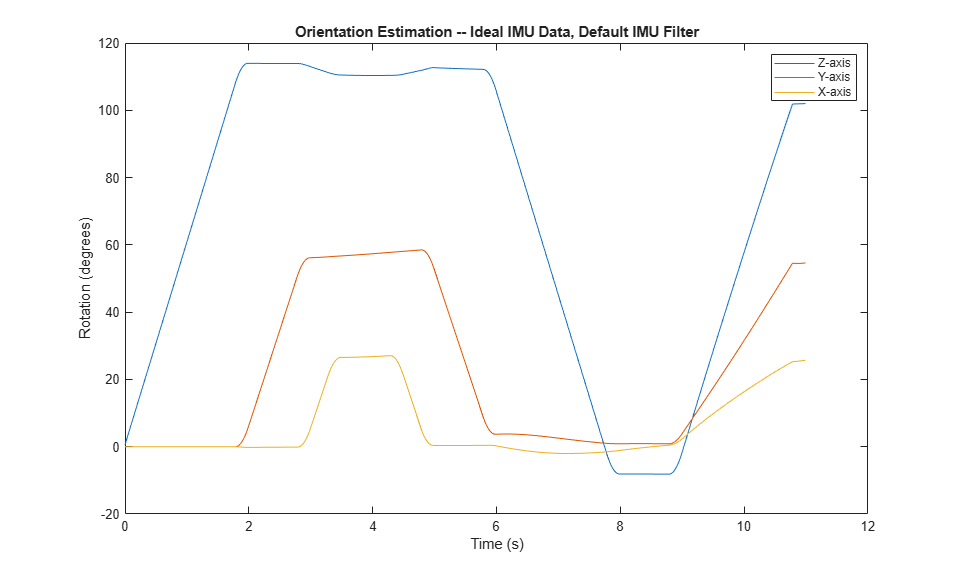Modify properties of your `imuSensor` to model real-world sensors. Run the loop again and plot the orientation estimate over time.

```IMU.Accelerometer = accelparams( ... 'MeasurementRange',19.62, ... 'Resolution',0.00059875, ... 'ConstantBias',0.4905, ... 'AxesMisalignment',2, ... 'NoiseDensity',0.003924, ... 'BiasInstability',0, ... 'TemperatureBias', [0.34335 0.34335 0.5886], ... 'TemperatureScaleFactor',0.02); IMU.Gyroscope = gyroparams( ... 'MeasurementRange',4.3633, ... 'Resolution',0.00013323, ... 'AxesMisalignment',2, ... 'NoiseDensity',8.7266e-05, ... 'TemperatureBias',0.34907, ... 'TemperatureScaleFactor',0.02, ... 'AccelerationBias',0.00017809, ... 'ConstantBias',[0.3491,0.5,0]); orientationDefault = zeros(numSamples,1,'quaternion'); for i = 1:numSamples [accelBody,gyroBody] = IMU(accNED(i,:),angVelNED(i,:),orientationNED(i,:)); orientationDefault(i) = aFilter(accelBody,gyroBody); end release(aFilter) figure(2) plot(t,eulerd(orientationDefault,'ZYX','frame')) xlabel('Time (s)') ylabel('Rotation (degrees)') title('Orientation Estimation -- Realistic IMU Data, Default IMU Filter') legend('Z-axis','Y-axis','X-axis') ```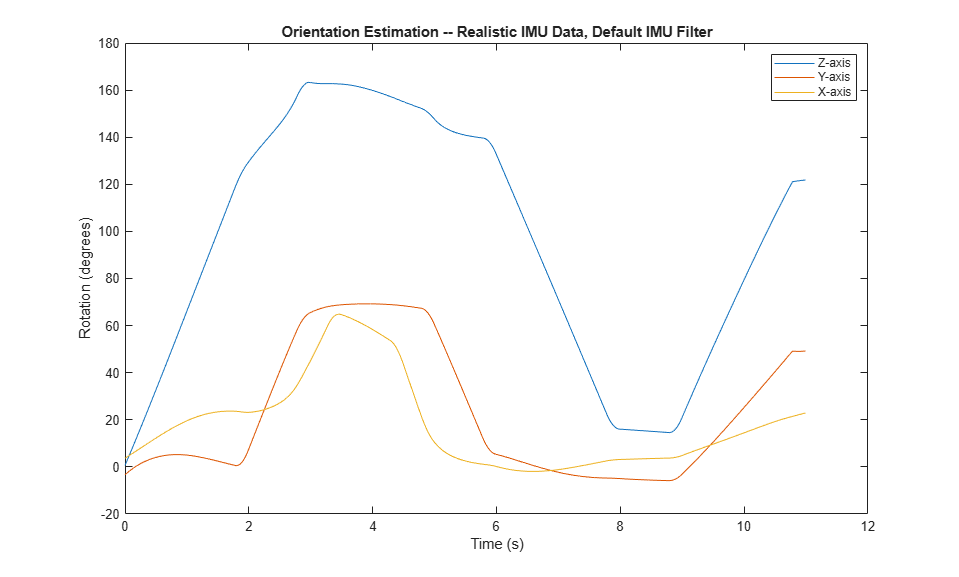The ability of the `imufilter` to track the ground-truth data is significantly reduced when modeling a realistic IMU. To improve performance, modify properties of your `imufilter` object. These values were determined empirically. Run the loop again and plot the orientation estimate over time.

```aFilter.GyroscopeNoise = 7.6154e-7; aFilter.AccelerometerNoise = 0.0015398; aFilter.GyroscopeDriftNoise = 3.0462e-12; aFilter.LinearAccelerationNoise = 0.00096236; aFilter.InitialProcessNoise = aFilter.InitialProcessNoise*10; orientationNondefault = zeros(numSamples,1,'quaternion'); for i = 1:numSamples [accelBody,gyroBody] = IMU(accNED(i,:),angVelNED(i,:),orientationNED(i,:)); orientationNondefault(i) = aFilter(accelBody,gyroBody); end release(aFilter) figure(3) plot(t,eulerd(orientationNondefault,'ZYX','frame')) xlabel('Time (s)') ylabel('Rotation (degrees)') title('Orientation Estimation -- Realistic IMU Data, Nondefault IMU Filter') legend('Z-axis','Y-axis','X-axis') ```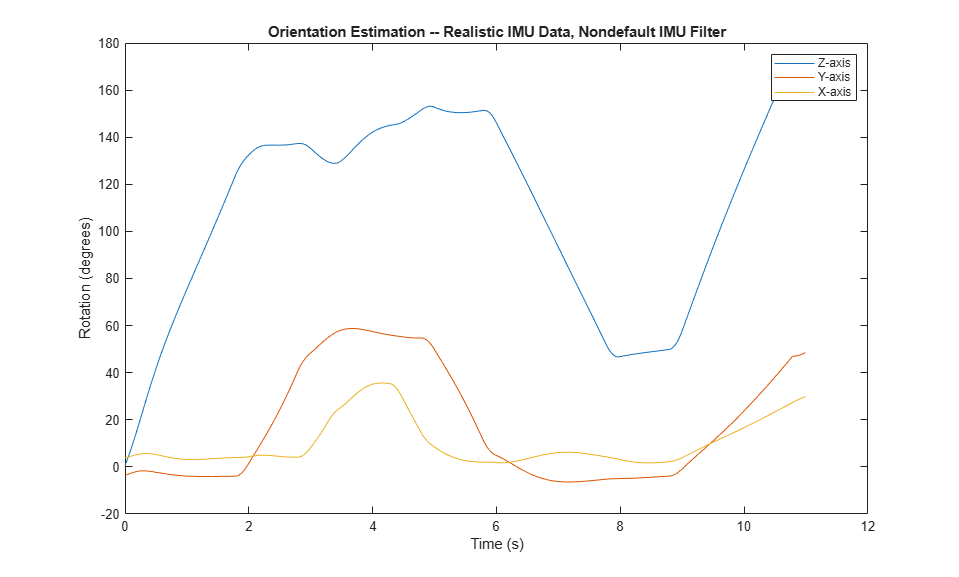To quantify the improved performance of the modified `imufilter`, plot the quaternion distance between the ground-truth motion and the orientation as returned by the `imufilter` with default and nondefault properties.

```qDistDefault = rad2deg(dist(orientationNED,orientationDefault)); qDistNondefault = rad2deg(dist(orientationNED,orientationNondefault)); figure(4) plot(t,[qDistDefault,qDistNondefault]) title('Quaternion Distance from True Orientation') legend('Realistic IMU Data, Default IMU Filter', ... 'Realistic IMU Data, Nondefault IMU Filter') xlabel('Time (s)') ylabel('Quaternion Distance (degrees)') ```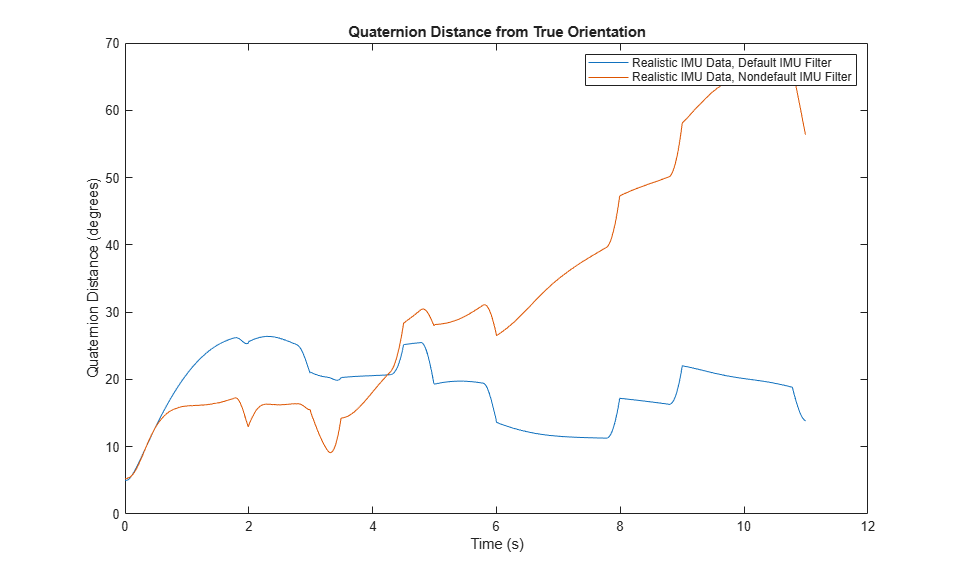expand all

## Extended Capabilities

### System Objects

#### Introduced in R2018b